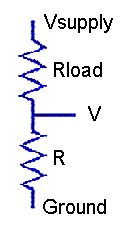DAPL Operating System | Processing Command

Module SENSORM :: DIVIDER

Convert measurements from a voltage divider network to determine resistance.

Syntax

DIVIDER( VIN, VS, RLOAD, [RCOEFF, LTMP,] [GAIN,] ROUT )

Parameters

VIN
Input measurements of divider voltage
FLOAT PIPE
VS
Nominal or measured excitation voltage driving divider network
FLOAT CONSTANT | FLOAT PIPE
Nominal or calibrated load resistance, ohms at 0 degrees C
FLOAT CONSTANT
RCOEFF
Temperature coefficient of resistance for load resistor
FLOAT CONSTANT
LTMP
Input pipe with load temperature measurements, degrees C
FLOAT PIPE
GAIN
Gain used to measure input signal VIN
FLOAT CONSTANT
ROUT
Output resistance measurements, in ohms
FLOAT PIPE

Description

The DIVIDER command converts voltage readings to measure an unknown resistance. The unknown device to be measured is placed in a voltage divider network with an accurately-known loading resistor. The divider network is driven by an accurately-regulated voltage source, and the voltages VIN are observed at the junction between the unknown element and load resistor. The results, in ohms, are returned through the ROUT pipe, one result per divider measurement input value.The input divider voltage measurements are received through the VIN pipe. These measurements have units of volts. By default, this command assumes that the voltage measurement are obtained using an amplifier gain of 1.0, but if you use a higher gain, specify it as the GAIN parameter.

If the supply voltage is very well regulated, you can measure it once and specify the value as a constant VS parameter. If you use the regulated voltage from the data acquisition processor for this excitation, you could specify 5.0 volts and omit the calibration measurement. If the excitation is subject to small but potentially relevant variations, make simultaneous voltage measurements of the supply voltage, in units of volts, and provide this second input stream to the DIVIDER command through the VS pipe, one supply voltage measurement per divider voltage reading.

Specify the loading resistor value RLOAD parameter in units of ohms. Sometimes a nominal resistor value is close enough, but for better results enter an accurately- measured value.

For processing each input value, the difference between the known excitation voltage source and the measured divider junction appears across the known loading resistor, hence the divider current can be computed. This known divider current passes through the unknown element to produce the measured voltage, so its resistance can be computed, producing the results placed into the ROUT pipe.

Examples

DIVIDER(IP2, 5.000, 10000.0, ROUT)

Read the voltage at the junction between a load resistance of exactly 10K ohms and an unknown resistive sensor when a 5.000 volt excitation voltage is applied across the divider network. The voltages across the resistive sensor element are measured with gain 1 and received from input sample channel pipe IPipe 2. The computed resistance values are reported in pipe ROUT.

DIVIDER(IP2, 4.968, 10008.0, 0.42, PAmb, 4.0, ROUT)

This example is the same as the previous configuration, except calibrated for maximum measurement accuracy. The pre-measured supply voltage level is 4.958 ohms is specified as a constant. The measured load resistor value is 10008 ohms. The nominal load resistance is observed to increase by 42 ohms over a 100 degree temperature swing, so a temperature coefficient 0.42 ohms per degrees C is specified. The measurements of divider voltage are captured using an amplifier gain of 4.0. The operating temperature is separately measured and provided in the PAmb pipe. The computations use a temperature-adjusted value of load resistance for each conversion.